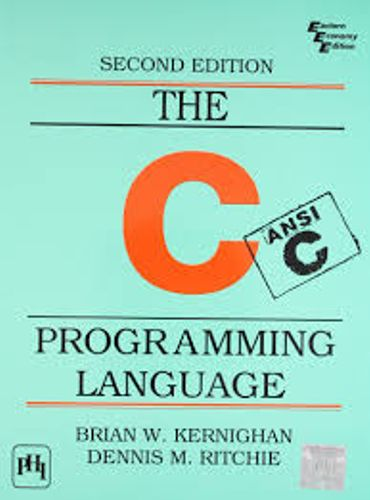# While loops

While loops are similar to for loops, but have less functionality. A while loop continues executing the while block as long as the condition in the while remains true. For example, the following code will execute exactly ten times:

```int n = 0;
while (n < 10) {
n++;
}
```

While loops can also execute infinitely if a condition is given which always evaluates as true (non-zero):

```while (1) {
/* do something */
}
```

### Loop directives

There are two important loop directives that are used in conjunction with all loop types in C - the `break` and `continue` directives.

The `break` directive halts a loop after ten loops, even though the while loop never finishes:

```int n = 0;
while (1) {
n++;
if (n == 10) {
break;
}
}
```

In the following code, the `continue` directive causes the `printf` command to be skipped, so that only even numbers are printed out:

```int n = 0;
while (n < 10) {
n++;

/* check that n is odd */
if (n % 2 == 1) {
/* go back to the start of the while block */
continue;
}

/* we reach this code only if n is even */
printf("The number %d is even.\n", n);
}
```

## Home Work

The `array` variable consists of a sequence of ten numbers. Inside the while loop, you must write two `if` conditions, which change the flow of the loop in the following manner (without changing the `printf` command):

• If the current number which is about to printed is less than 5, don't print it.
• If the current number which is about to printed is greater than 10, don't print it and stop the loop.

Notice that if you do not advance the iterator variable `i` and use the `continue` derivative, you will get stuck in an infinite loop.

Click on image to Download C Programming Language Book :